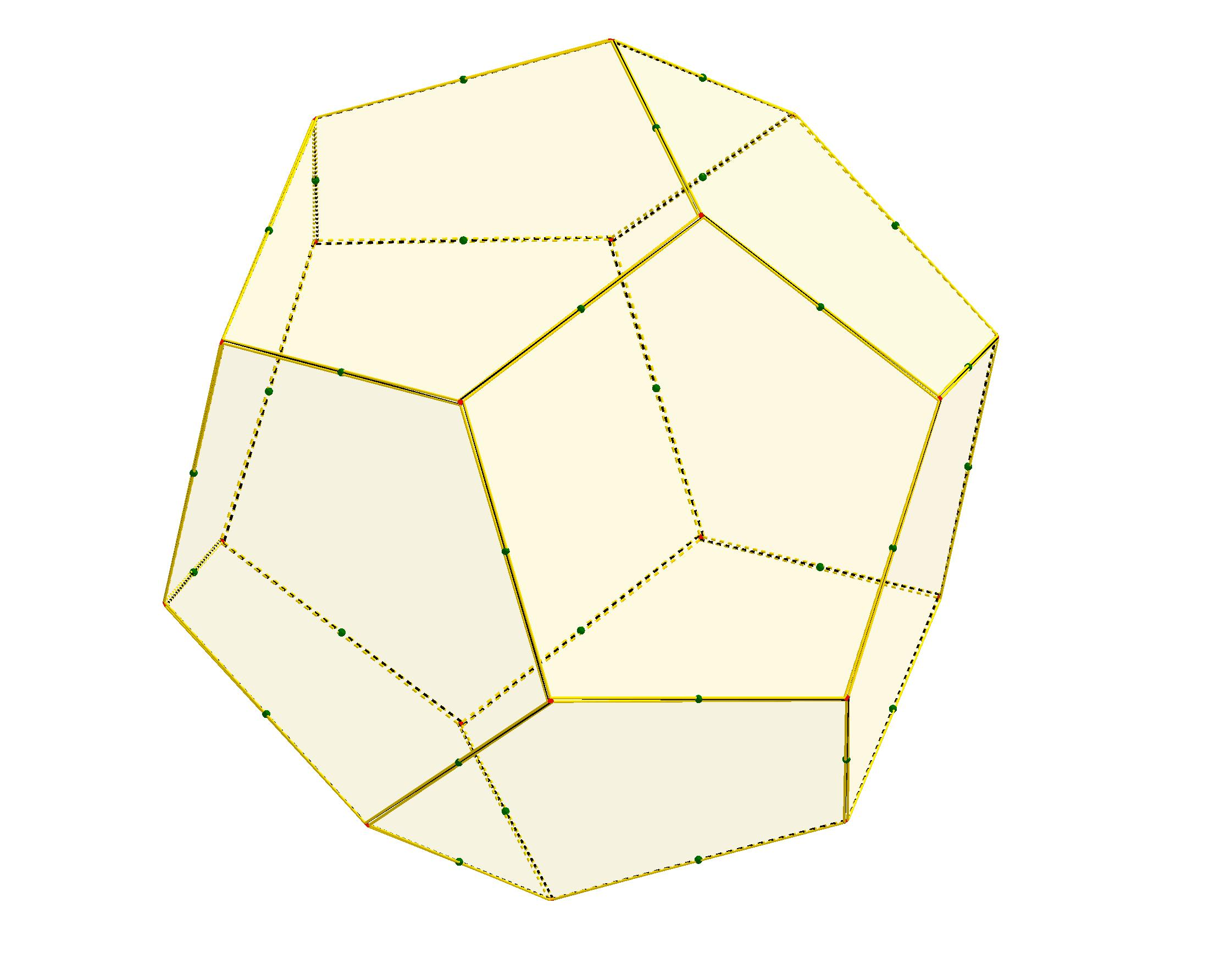09 Mar

As mentioned and illustrated in the post on the Anticos, it’s possible to inscribe an icosahedron in a cube. (In this case, that technically means that given a cube, you can choose two points on each face of the cube such that the convex hull of the resulting set of twelve points is a regular icosahedron.)

But why should this be so? To see this, it’s easiest to start with a regular dodecahedron, say with unit edge length. Notice the interesting pattern of the blue face diagonals in this diagram:

Clearly all of the blue segments are the same length, since each is a diagonal of a regular pentagon with unit edge length. Moreover, since rotating the outer dodecahedron a half turn around the axis joining the midpoints of any two opposite red edges brings the dodecahedron back to itself, it must do the same to the blue figure. These facts (single edge length, three perpendicular twofold axes of symmetry) are enough to guarantee that the blue figure is precisely a cube.

Moreover, it’s also clear from the diagram that the red edges are parallel to the faces of this blue cube. (By the symmetries highlighted in the last paragraph, both endpoints of a red edge are the same distance from the nearest face plane of the cube.) Therefore, if we expanded the cube slightly, each red edge would lie in a face of the cube. In other words, a regular dodecahedron has a property very similar to the one we are looking for concerning the icosahedron. (Which begs the question of what the Antidodec would look like — an S∞ project for another day!)

We want to transfer this property to the icosahedron. Now, the dodecahedron is the dual of the icosahedron. And one, perhaps slightly less well-known, way of passing from a regular polyhedron to its dual is to rotate each edge a quarter turn around the axis connecting its midpoint to the center of the polyhedron:Notice that during this transformation, each of the edges remains in the plane perpendicular to that axis. Therefore, twelve of the vertices of an icosahedron lie on the surface of a cube, two on each face. Since an icosahedron only has twelve vertices, it is inscribed in a cube.